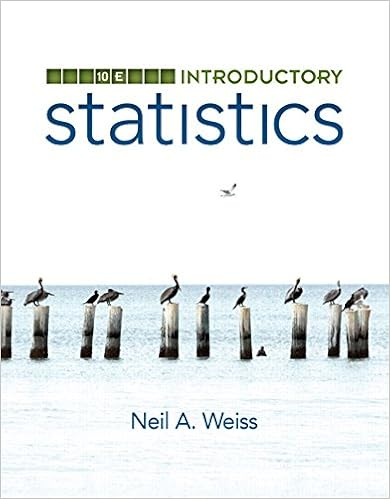# 9 in a fish farm a population of fish is introduced

• Homework Help
• jameshillier
• 6
• 100% (2) 2 out of 2 people found this document helpful

This preview shows page 6 out of 6 pages.

9. (****) In a fish farm, a population of fish is introduced into a pond and harvested regularly.A model for the rate of change of fish population is given by the (differential) equationdPdt=r01-P(t)PcP(t)-βP(t)wherer0is the birth rate of the fish,Pcis the maximum population that the pond cansustain (called thecarrying capacity), andβis the percentage of the population that isharvested.1. What value ofdP/dtcorresponds to a stable population?
2. If the pond can sustain 10,000 fish, the birth rate is 5%, and the harvesting rate is 4%,find the stable population level.
3. What happened ifβis raised to 5%?5
##### We have textbook solutions for you!The document you are viewing contains questions related to this textbook.
Chapter 2 / Exercise 13
Essential Calculus
StewartExpert VerifiedBrowse all Textbook Solutions
End of preview. Want to read all 6 pages?

Course Hero member to access this document

Term
Spring
Professor
LAMB
Tags
Math, lim, Natural logarithm, Logarithm
##### We have textbook solutions for you!
The document you are viewing contains questions related to this textbook.The document you are viewing contains questions related to this textbook.
Chapter 2 / Exercise 13
Essential Calculus
StewartExpert Verified
•••